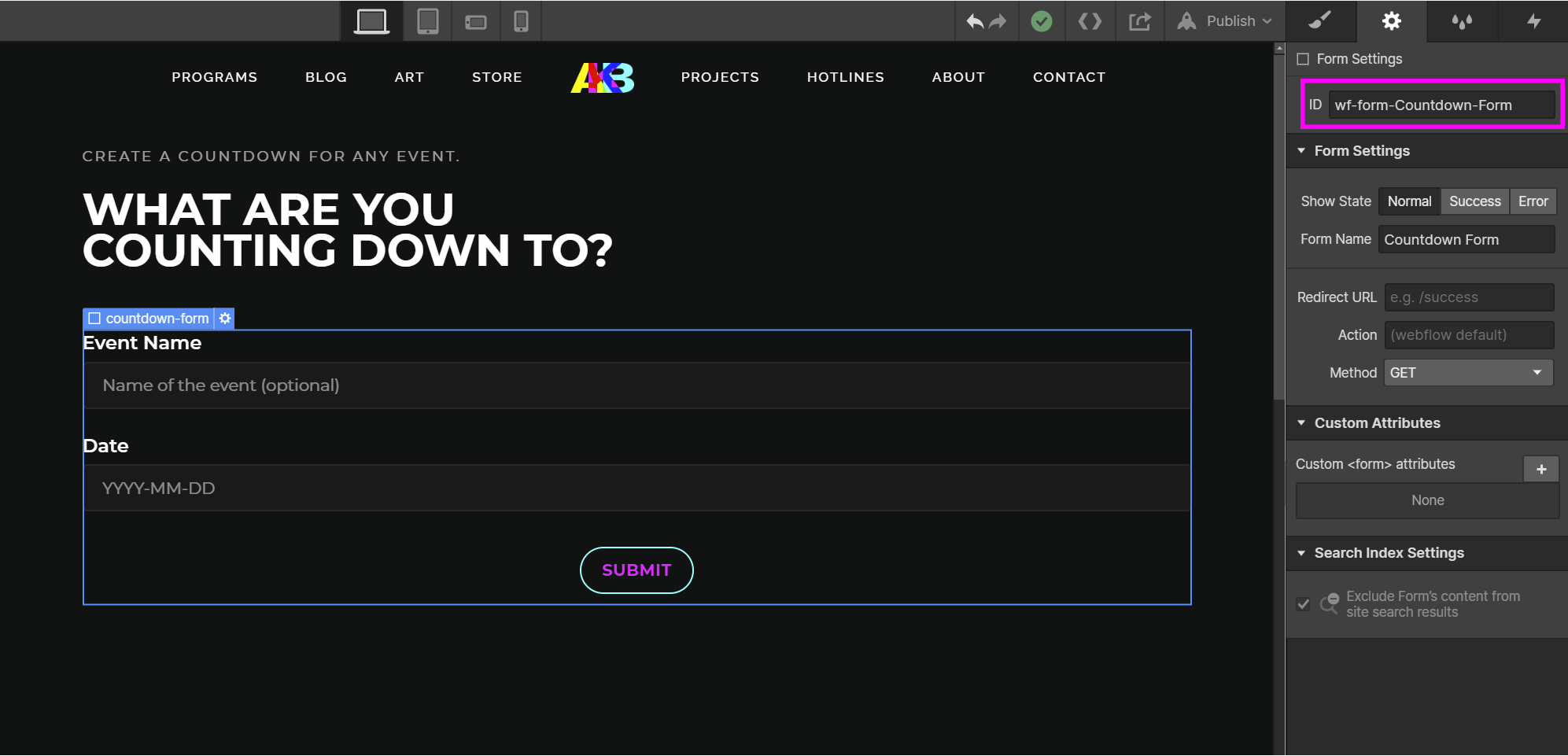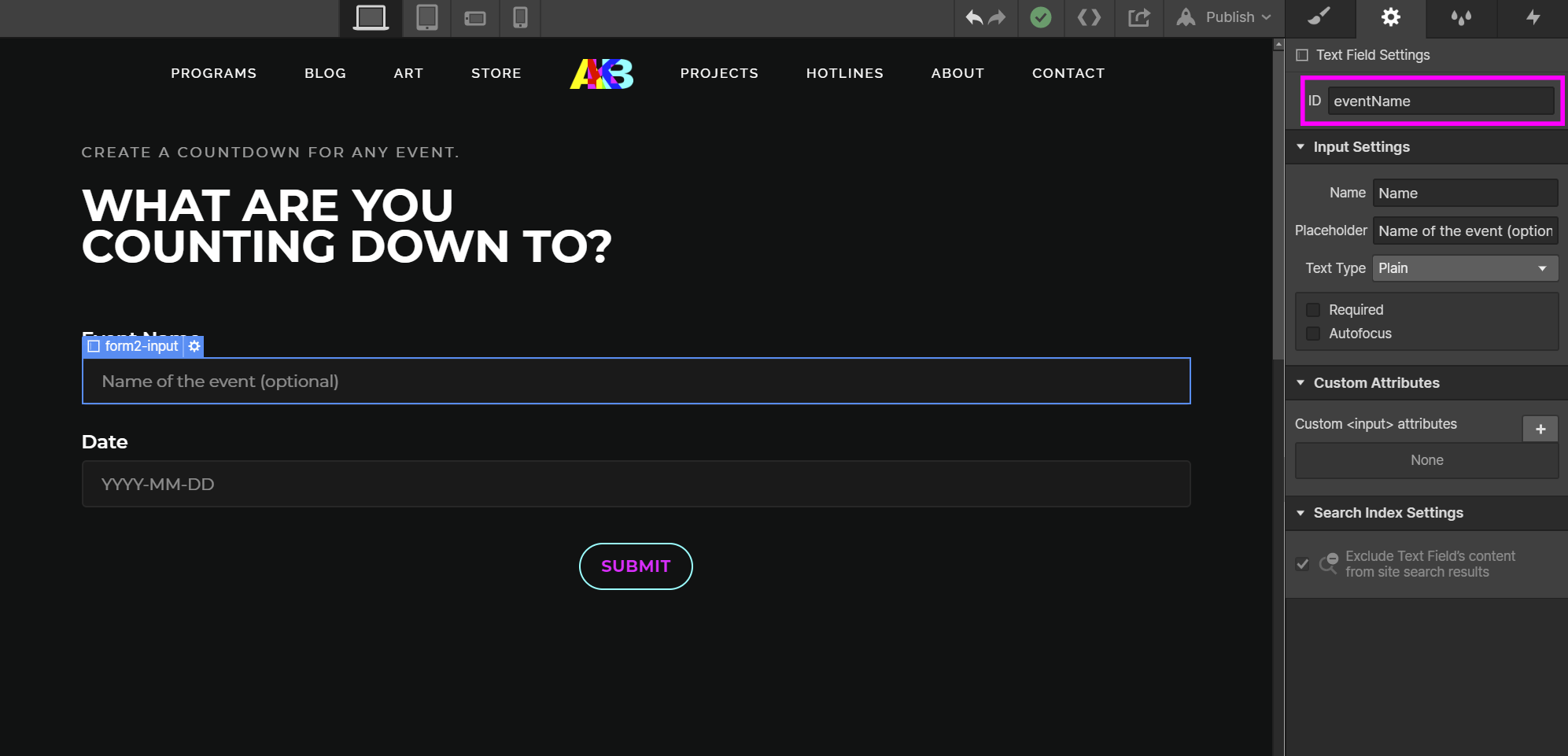create a countdown for any event.

## what are you counting down to?

Oops! Something went wrong while submitting the form. That's on us. Try refreshing the page.
1. Create the form using Webflow's built-in form block.
Make sure to set the ID of the heading, the form, its text fields and its success message (it will be necessary later)Click the element you want to set an ID for, navigate to its settings and enter a value in for its ID. I showed some examples above, but I also had to set IDs for the heading and the success message to manipulate them in the script.
2. Calculate the current time remaining until the date entered in the form.
The IDs are used in this step. The form ID is used to create the variable "countdownForm" which will be used to add an event listener to the form and the field ID is used to get the value of the data entered in the eventDate field.
``````<script type="text/javascript">

let countdownForm = document.getElementById("wf-form-Countdown-Form");

// initializing variables
let sec;
let min;
let hour;
let day;

function countdown(){

// initailizing to be used for the current time
let now = new Date();

// taking input from the form to create event date object
let eventDateData = document.getElementById("eventDate").value;
let eventDate = new Date(eventDateData);

// convert the dates to millseconds to find the difference between the two dates
let currentTime = now.getTime();
let eventTime = eventDate.getTime();

let remTime = eventTime - currentTime;

// converting milliseconds to seconds, minutes, hours and days
sec = Math.floor(remTime / 1000);
min = Math.floor(sec / 60);
hour = Math.floor(min / 60);
day = Math.floor(hour / 24);

hour %= 24;
min %= 60;
sec %= 60;
}
``````
4. Create a function to display the remaining time in the success message of the form.
This function takes the event name and the remaining time in hours, minutes and seconds as parameters. The IDs are used in this step. The form heading ID is used to set the heading to the event name and the success message ID is used to set the success message to the countdown time.
``````    function display(eventName, h, m, s){

// set eventName to "event" if it's not set
if (eventName == ""){
eventName = "event";
}

let timeRemaining = [day + " days",
hour + " hours",
min + " minutes",
sec + " seconds"].join("\n");

// copying the event name to the form's heading
document.getElementById("form-heading").innerText = "countdown to " + eventName;
document.getElementById("form-heading").textContent = "Countdown to " + eventName;

// adding styling to the success message and copying the time to it
let successMsg = document.getElementById("success-msg");
successMsg.style.whiteSpace = "pre";
successMsg.innerText = timeRemaining;
successMsg.textContent = timeRemaining;
}
``````
5. Create a wrapper function to call the display function.
The IDs are used in this step. The field ID is used to get the value of the data entered in the eventName field. The countdownForm variable created in Step 2 is used to add an event listener that executes the script when the form is submitted.
``````    function startDisplay(){
let eventNameData = document.getElementById("eventName").value;
setInterval( function(){ countdown(); display(eventNameData, hour, min, sec); }, 1000);
}
</script>
``````
Your entire script should look like:
``````<script type="text/javascript">

let countdownForm = document.getElementById("wf-form-Countdown-Form");

// initializing variables
let sec;
let min;
let hour;
let day;

function countdown(){

// initailizing to be used for the current time
let now = new Date();

// taking input from the form to create event date object
let eventDateData = document.getElementById("eventDate").value;
let eventDate = new Date(eventDateData);

// convert the dates to millseconds to find the difference between the two dates
let currentTime = now.getTime();
let eventTime = eventDate.getTime();

let remTime = eventTime - currentTime;

// converting milliseconds to seconds, minutes, hours and days
sec = Math.floor(remTime / 1000);
min = Math.floor(sec / 60);
hour = Math.floor(min / 60);
day = Math.floor(hour / 24);

hour %= 24;
min %= 60;
sec %= 60;
}

function display(eventName, h, m, s){

// set eventName to "event" if it's not set
if (eventName == ""){
eventName = "event";
}

let timeRemaining = [day + " days",
hour + " hours",
min + " minutes",
sec + " seconds"].join("\n");

// copying the event name to the form's heading
document.getElementById("form-heading").innerText = "countdown to " + eventName;
document.getElementById("form-heading").textContent = "Countdown to " + eventName;

// adding styling to the success message and copying the time to it
let successMsg = document.getElementById("success-msg");
successMsg.style.whiteSpace = "pre";